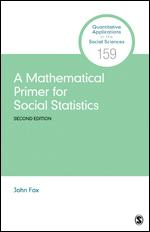# A Mathematical Primer for Social Statistics

2nd Edition

John Fox
eISBN-13: 9781071833230## eBook Features

Rent or Buy from \$ 16.00 USD
Note: We do not guarantee supplemental material with textbooks (e.g. CD's, Music, DVD's, Access Code, or Lab Manuals)

## Additional Book Details

A Mathematical Primer for Social Statistics, Second Edition presents mathematics central to learning and understanding statistical methods beyond the introductory level: the basic "language" of matrices and linear algebra and its visual representation, vector geometry; differential and integral calculus; probability theory; common probability distributions; statistical estimation and inference, including likelihood-based and Bayesian methods. The volume concludes by applying mathematical concepts and operations to a familiar case, linear least-squares regression. The Second Edition pays more attention to visualization, including the elliptical geometry of quadratic forms and its application to statistics. It also covers some new topics, such as an introduction to Markov-Chain Monte Carlo methods, which are important in modern Bayesian statistics. A companion website includes materials that enable readers to use the R statistical computing environment to reproduce and explore computations and visualizations presented in the text. The book is an excellent companion to a "math camp" or a course designed to provide foundational mathematics needed to understand relatively advanced statistical methods.

ISBNs 9781071833209, 9781071833209, 1071833235, 9781071833230 English 240 2nd